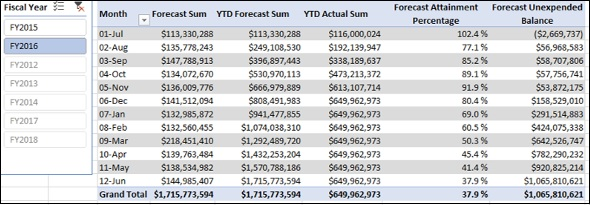# Forecast Measures and Analysis

You can use Forecast measures to analyze the finance data and help an organization make necessary adjustments in its goals and targets for the year, to align the company’s performance to the changing business requirements.

You need to update the forecasts regularly to keep up with the changes. You can then compare the most recent forecast to the budget for the rest of the period in the financial year so that the company can make the required adjustments to meet the business changes.

At any time during a financial year, you can calculate the following −

### Forecast Attainment %

Forecast Attainment % is the percentage of the forecast sum that you have spent to date, i.e.

Forecast Attainment % = YTD Actual Sum/YTD Forecast Sum

### Forecast Unexpended Balance

Forecast Unexpended Balance is the Forecast Sum remaining after the actual expenses, i.e

Forecast Unexpended Balance = YTD Forecast Sum – YTD Actual Sum

Budget Adjustment is the adjustment in the budget sum an organization needs to make (an increase or decrease) based on the forecast.

Budget Adjustment = Forecast Unexpended Balance - Unexpended Balance

The budget needs to be increased if the resulting value is positive. Otherwise, it can be adjusted for some other purpose.

### Creating Forecast Attainment Percentage Measure

You can create Forecast Attainment Percentage measure as follows −

Forecast Attainment Percentage:= IF([YTD Forecast Sum], [YTD Actual Sum]/[YTD Forecast Sum], BLANK())

### Creating Forecast Unexpended Balance Measure

You can create Forecast Unexpended Balance measure as follows −

Forecast Unexpended Balance:=[YTD Forecast Sum]-[YTD Actual Sum]

You can create Budget Adjustment measure as follows −

## Analyzing Data with Forecast Measures

Create a Power PivotTable as follows −

• Add Month from Date table to Rows.

• Add the measures Budget Sum, YTD Budget Sum, YTD Actual Sum, Budget Attainment % and Unexpended Balance from Finance Data table to Values.

• Insert a Slicer on Fiscal Year.

• Select FY2016 in the Slicer.